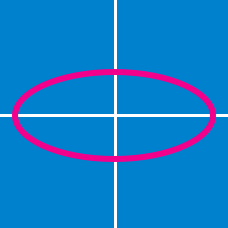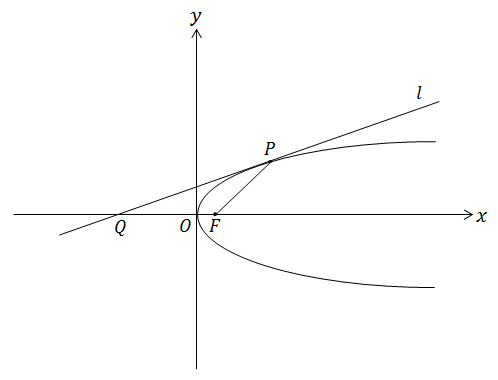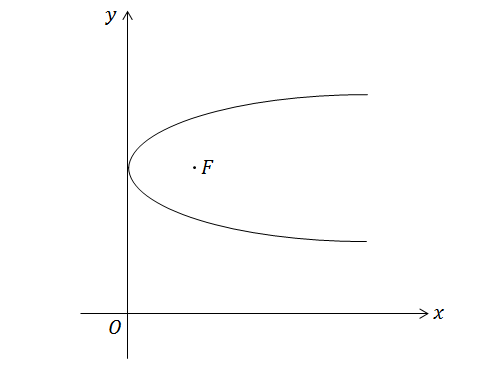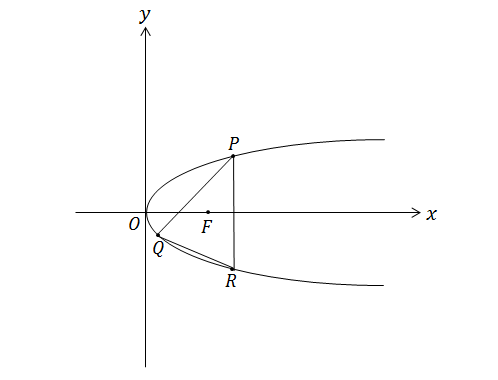Geometry

# Conics - Parabola - Focus and DirectrixThe above diagram shows a point $P$ on a parabola $y^2=12x$ with focus $F$. If $l$ is a tangent line to the parabola at point $P$, the $x$-intercept of $l$ is $Q$, and $\angle FPQ=36^\circ,$ what is $\angle PFQ$ in degrees?

Note: The above figure is not drawn to scale.The above diagram shows a parabola with vertex on the $y$-axis, and directrix parallel to the $y$-axis. If the focus of the parabola is $F=(4,6)$, what is the equation for the parabola?

Note: The above diagram is not drawn to scale.

If $y=ax+b$ is the equation for a line with slope $6$ that is a tangent line of the parabola $y^2=12x$, what is $a+b?$

Identify the focus and directrix of the parabola given by $x =-\frac{1}{28}y^{2}.$In the diagram above, points $P, Q,$ and $R$ lie on parabola $y^2=60x.$ If the center of gravity of $\triangle PQR$ is the focus $F$ of the parabola, what is the sum of the lengths $\overline{PF}, \overline{QF},$ and $\overline{RF}?$

Note: The figure above is not drawn to scale.

×